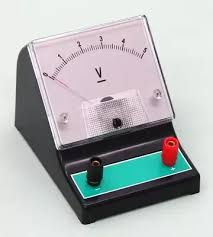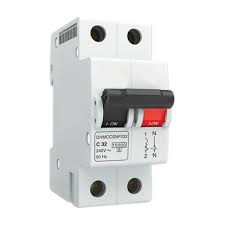# Difference between voltage and current

## Difference between voltage and current

What is Difference between current and voltage. In the electrical you will find a word or a term that is often called The word is electric current and voltage. Well here I will tell you about the difference between  electric voltage  and electric current and how to measure the voltage and current of electricity.

### The difference between electric current and voltage is

What are current and voltage difference
In this post I will tell you about the electric current and electric voltage.

#### Electric voltage definition

Electrical voltage is the potential difference between the two poles. The voltage size is expressed in volts or we can say that voltage is measured in volts.

Examples of daily life we will often encounter 220V AC voltage.Voltmeter

If in the world of automation you will find 12V DC voltage is the motorcycle or car battery voltage and in electronics we will use 12V DC supply and so on.The tool for measuring the voltage is called a voltmeter.
The Voltage is measured in Volt.

Difference between AC and DC current
How to install home lighting

Here the meaning of electric current is the amount of electric charge flowing per unit time in a conductor.
When the flow of electric charge is more the greater the electrical current generated.

The size of the electric current is expressed in amperes. But because the ampere is a size that is too large usually large electric current is also expressed in the size of milli amperes (mA) and micro amperes (uA).Ammeter

Tool to measure the magnitude of electric current is called ampere meter.

volt meter and ampere meter and MCB are shown in fig.

electric current definition
electric voltage definition

In electrical circuit if the flow of electricity that is large enough to cause heat and even cause a fire
Meanwhile, the battery the greater the flow of electricity that will result in the battery run out quickly.

In electrical circuits in homes to avoid excessive electricity is usually installed a tool called MCB (miniature circuit breaker) or can also use a fuse.MCB

what is conductor and insulator
difference b/w shell type and core type transformer

how to measure current and power voltage

for how to measure the current and voltage you can use voltmeter but before making the measurement you must know first of the type AC or DC Because the measurement using different types of equipment.

For mounting voltmeter installed in parallel while for mounting ampere meter mounted in series.

electric current and voltage definition